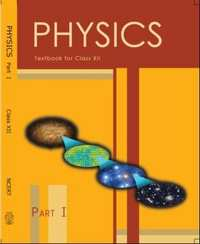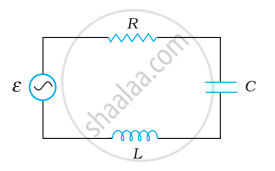# NCERT solutions for Class 12 Physics Textbook chapter 7 - Alternating Current [Latest edition]

#### Chapters## Solutions for Chapter 7: Alternating Current

Below listed, you can find solutions for Chapter 7 of CBSE, Karnataka Board PUC NCERT for Class 12 Physics Textbook.

Exercise
Exercise [Page 266]

### NCERT solutions for Class 12 Physics Textbook Chapter 7 Alternating Current Exercise [Page 266]

Exercise | Q 7.01 | Page 266

A 100 Ω resistor is connected to a 220 V, 50 Hz ac supply.

1. What is the rms value of current in the circuit?
2. What is the net power consumed over a full cycle?
Exercise | Q (b) | Page 266

The rms value of current in an ac circuit is 10 A. What is the peak current?

Exercise | Q (a) | Page 266

The peak voltage of an ac supply is 300 V. What is the rms voltage?

Exercise | Q 7.03 | Page 266

A 44 mH inductor is connected to 220 V, 50 Hz ac supply. Determine the rms value of the current in the circuit.

Exercise | Q 7.04 | Page 266

A 60 µF capacitor is connected to a 110 V, 60 Hz ac supply. Determine the rms value of the current in the circuit.

Exercise | Q 7.05 | Page 266

What is the net power absorbed by each circuit over a complete cycle. Explain your answer.

Exercise | Q 7.06 | Page 266

Obtain the resonant frequency ωr of a series LCR circuit with L = 2.0 H, C = 32 µF and R = 10 Ω. What is the Q-value of this circuit?

Exercise | Q 7.07 | Page 266

A charged 30 µF capacitor is connected to a 27 mH inductor. What is the angular frequency of free oscillations of the circuit?

Exercise | Q 7.08 | Page 266

Suppose the initial charge on the capacitor is 6 mC. What is the total energy stored in the circuit initially? What is the total energy at later time?

Exercise | Q 7.09 | Page 266

A series LCR circuit with R = 20 Ω, L = 1.5 H and C = 35 µF is connected to a variable-frequency 200 V ac supply. When the frequency of the supply equals the natural frequency of the circuit, what is the average power transferred to the circuit in one complete cycle?

Exercise | Q 7.1 | Page 266

A radio can tune over the frequency range of a portion of MW broadcast band: (800 kHz to 1200 kHz). If its LC circuit has an effective inductance of 200 µH, what must be the range of its variable capacitor?

[Hint: For tuning, the natural frequency i.e., the frequency of free oscillations of the LC circuit should be equal to the frequency of the radio wave.]

Exercise | Q 7.11 | Page 266

Figure shows a series LCR circuit connected to a variable frequency 230 V source. L = 5.0 H, C = 80 µF, R = 40 Ω.(a) Determine the source frequency which drives the circuit in resonance.

(b) Obtain the impedance of the circuit and the amplitude of current at the resonating frequency.

(c) Determine the rms potential drops across the three elements of the circuit. Show that the potential drop across the LC combination is zero at the resonating frequency.

## Solutions for Chapter 7: Alternating Current

Exercise## NCERT solutions for Class 12 Physics Textbook chapter 7 - Alternating Current

Shaalaa.com has the CBSE, Karnataka Board PUC Mathematics Class 12 Physics Textbook CBSE, Karnataka Board PUC solutions in a manner that help students grasp basic concepts better and faster. The detailed, step-by-step solutions will help you understand the concepts better and clarify any confusion. NCERT solutions for Mathematics Class 12 Physics Textbook CBSE, Karnataka Board PUC 7 (Alternating Current) include all questions with answers and detailed explanations. This will clear students' doubts about questions and improve their application skills while preparing for board exams.

Further, we at Shaalaa.com provide such solutions so students can prepare for written exams. NCERT textbook solutions can be a core help for self-study and provide excellent self-help guidance for students.

Concepts covered in Class 12 Physics Textbook chapter 7 Alternating Current are LC Oscillations, Reactance and Impedance, Peak and Rms Value of Alternating Current Or Voltage, Alternating Currents, Different Types of AC Circuits: AC Voltage Applied to an Inductor, Different Types of AC Circuits: AC Voltage Applied to a Series LCR Circuit, Different Types of AC Circuits: AC Voltage Applied to a Capacitor, Representation of AC Current and Voltage by Rotating Vectors - Phasors, Different Types of AC Circuits: AC Voltage Applied to a Resistor, Alternating Currents and Direct Currents, Forced Oscillations and Resonance, Transformers, Power in AC Circuit: the Power Factor.

Using NCERT Class 12 Physics Textbook solutions Alternating Current exercise by students is an easy way to prepare for the exams, as they involve solutions arranged chapter-wise and also page-wise. The questions involved in NCERT Solutions are essential questions that can be asked in the final exam. Maximum CBSE, Karnataka Board PUC Class 12 Physics Textbook students prefer NCERT Textbook Solutions to score more in exams.

Get the free view of Chapter 7, Alternating Current Class 12 Physics Textbook additional questions for Mathematics Class 12 Physics Textbook CBSE, Karnataka Board PUC, and you can use Shaalaa.com to keep it handy for your exam preparation.